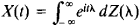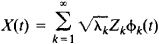# Spectral Resolution of a Random Function

## Spectral Resolution of a Random Function

an expansion of a random function, in particular, of a stochastic process, in a series or integral of some special system of functions where the coefficients of the expansion are mutually uncorrelated random variables.

The best known class of spectral resolutions of random functions consists of representations of stationary stochastic processes X(t) in the form of the Fourier-Stieltjes integralwhere Z(λ) is a random function with uncorrelated increments. The existence of this spectral resolution shows that a stationary stochastic process can always be regarded as the superposition of mutually uncorrelated harmonic oscillations of distinct frequencies with random phases and amplitudes. An analogous form of spectral resolution but with harmonic oscillations replaced by n-dimensional plane waves, holds for homogeneous random fields in n-dimensional space.

A further type of spectral resolution of random functions involves a stochastic process X(t) defined on a closed interval of the axis or, more generally, a random function X(t) defined on a bounded region of n-dimensional space. In this type, X(t) is expanded in a series of the formHere, φk(t) and λk are the eigenfunctions and eigenvalues of an integral operator in a function space with kernel equal to the correlation function of the stochastic process or random function X(t), and Zk, k = 1, 2, . . . , is a sequence of pairwise uncorrelated random variables with unit variance.

A special form of spectral resolution holds for homogeneous and isotropic random fields in Euclidean spaces and for homogeneous fields in spaces with a transformation group different from that of Euclidean space.

### REFERENCES

Iaglom, A. M. “Spektral’nye predstavleniia dlia razlichnykh klassov sluchainykh funktsii.” In Trudy 4-go Vsesoiuznogo matematiches-kogo s”ezda, vol. 1. Leningrad, 1963. Pages 250–73.
Gikhman, I. I., and A. V. Skorokhod. Teoriia sluchainykh protsessov, vol. 1. Moscow, 1971.

A. M. IAGLOM

Site: Follow: Share:
Open / Close